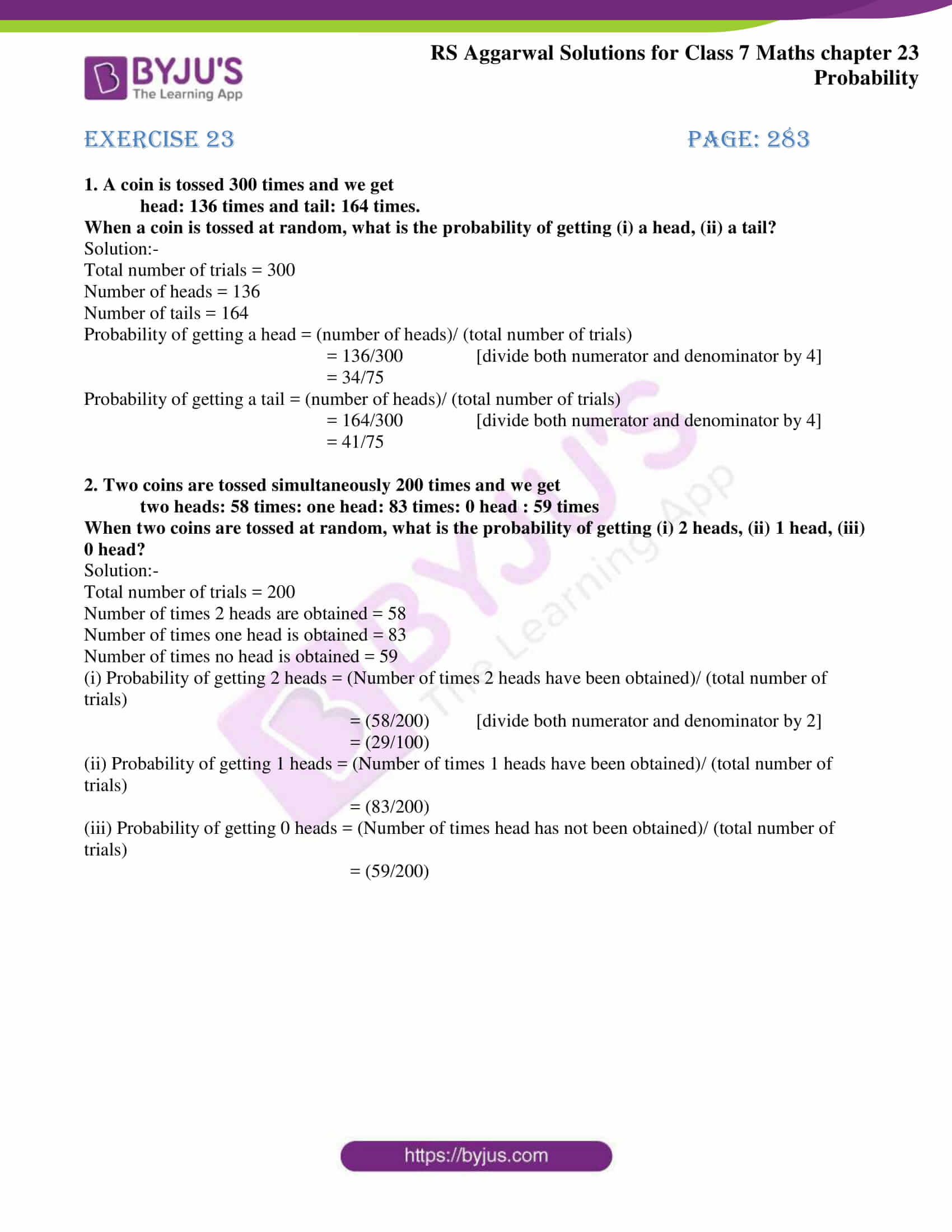# RS Aggarwal Solutions for Class 7 Maths Chapter 23 Probability

RS Aggarwal Solutions for Class 7 Maths Chapter 23 – Probability are available here. Maths is a subject which requires understanding and reasoning skills accompanied by logic. Along with this, it also requires students to practice Maths regularly. Students of Class 7 are suggested to solve RS Aggarwal Class 7 Solutions to strengthen their fundamentals and be able to solve questions that are usually asked in the examination.

The primary aim is to help students understand and crack these problems. We at BYJU’S have prepared the RS Aggarwal Solutions for Class 7 Maths Chapter 23 wherein problems are solved step by step with in-depth explanations. Download pdf of Class 7 Chapter 23 in their respective links.

## Download PDF of RS Aggarwal Solutions for Class 7 Maths Chapter 23 – Probability## Exercise 23 Page: 283

1. A coin is tossed 300 times and we get

head: 136 times and tail: 164 times.

When a coin is tossed at random, what is the probability of getting (i) a head, (ii) a tail?

Solution:-

Total number of trials = 300

Number of tails = 164

Probability of getting a head = (number of heads)/ (total number of trials)

= 136/300 [divide both numerator and denominator by 4]

= 34/75

Probability of getting a tail = (number of heads)/ (total number of trials)

= 164/300 [divide both numerator and denominator by 4]

= 41/75

2. Two coins are tossed simultaneously 200 times and we get

When two coins are tossed at random, what is the probability of getting (i) 2 heads, (ii) 1 head, (iii) 0 head?

Solution:-

Total number of trials = 200

Number of times 2 heads are obtained = 58

Number of times one head is obtained = 83

Number of times no head is obtained = 59

(i) Probability of getting 2 heads = (Number of times 2 heads have been obtained)/ (total number of trials)

= (58/200) [divide both numerator and denominator by 2]

= (29/100)

(ii) Probability of getting 1 heads = (Number of times 1 heads have been obtained)/ (total number of trials)

= (83/200)

(iii) Probability of getting 0 heads = (Number of times head has not been obtained)/ (total number of trials)

= (59/200)

## RS Aggarwal Solutions for Class 7 Maths Chapter 23 – Probability

Chapter 23 – Probability contains 1 exercise and the RS Aggarwal Solutions available on this page provide solutions to the questions present in the exercises. Now, let us have a look at some of the concepts discussed in this chapter.

• Some Terms Related to Probability
• Empirical Probability

### Chapter Brief of RS Aggarwal Solutions for Class 7 Maths Chapter 23 – Probability

RS Aggarwal Solutions for Class 7 Maths Chapter 23 – Probability. The words ‘most probably’, ‘chance’, doubt, etc., indicate uncertainty or probability of occurrence of an event. Though probability started with gambling, it is now used extensively in diverse fields such as, commerce, biological science, weather forecasting, etc.